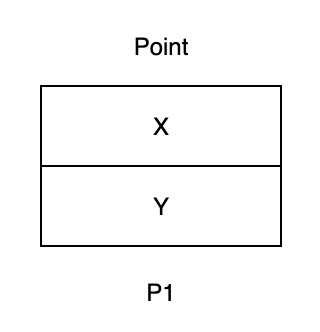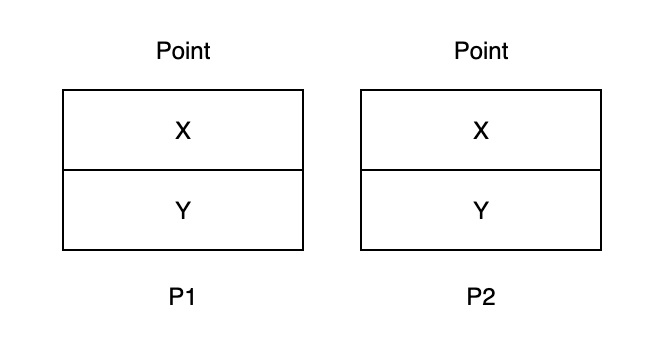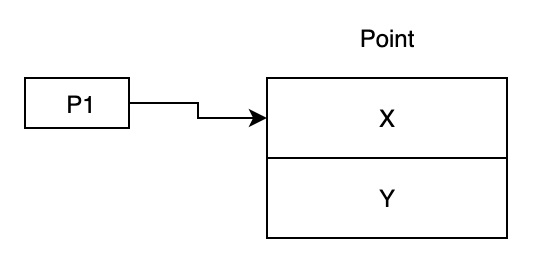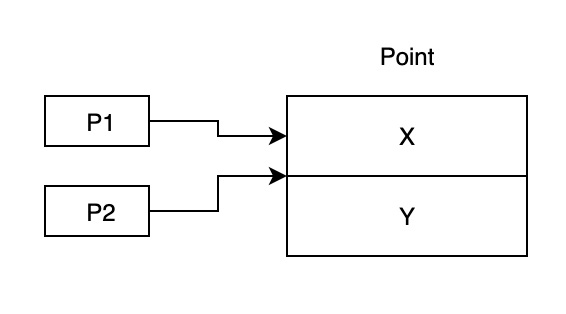# Explain and contrast value types and reference types in C#

In general, all types in C# can be divided into two main categories − value types and reference types. Let's look at each type in detail.

## Value Types

Variables of value types directly contain their data. Each variable has its own copy of the data. Hence it is impossible for a variable of value type to modify another object.

A value type can be one of the following types −

• all numeric types, e.g., int, float, and double
• char and the bool types
• struct type or
• enumeration type.

A value type simple contains the value. For example, the integer type contains the actual number, and not a pointer to the number, like reference types.

To create a custom value type, you can use a struct. For example −

public struct Point
{
public int X;
public int Y;
}
var p1 = new Point();

In memory, an instance of a Point is represented as follows −When you assign a variable of value type to another variable, the assignment operation creates a copy of the value being assigned. For example,

Point p2 = p1;## Reference Types

Variables of reference types store a reference to their objects. It's possible for two different variables to hold a reference to the same object. Any changes one variable makes to the object are visible to the second variable.

A reference type comprises of the following types −

• String
• Class
• Array
• Delegate
• Interface

For example, you can create a class, which is a reference type as follows −

public class Point{
public int X;
public int Y;
}
var p1 = new Point();When you assign a variable of reference type to another variable, the assignment operation simply copies the reference, and not the actual object.

Point p2 = p1;## Example

Live Demo

using System;
class Program{
static void Main(){
var p1 = new Point { X = 10 };
Point p2 = p1;
p2.X = 20;
Console.WriteLine("Value Type");
Console.WriteLine(p1.X);
Console.WriteLine(p2.X);
var u1 = new User { Age = 10 };
User u2 = u1;
u2.Age = 20;
Console.WriteLine("Reference Type");

Console.WriteLine(u1.Age);
Console.WriteLine(u2.Age);
}
}
public struct Point{
public int X;

public int Y;
}
public class User{
public int Age { get; set; }
}

## Output

Value Type
10
20
Reference Type
20
20